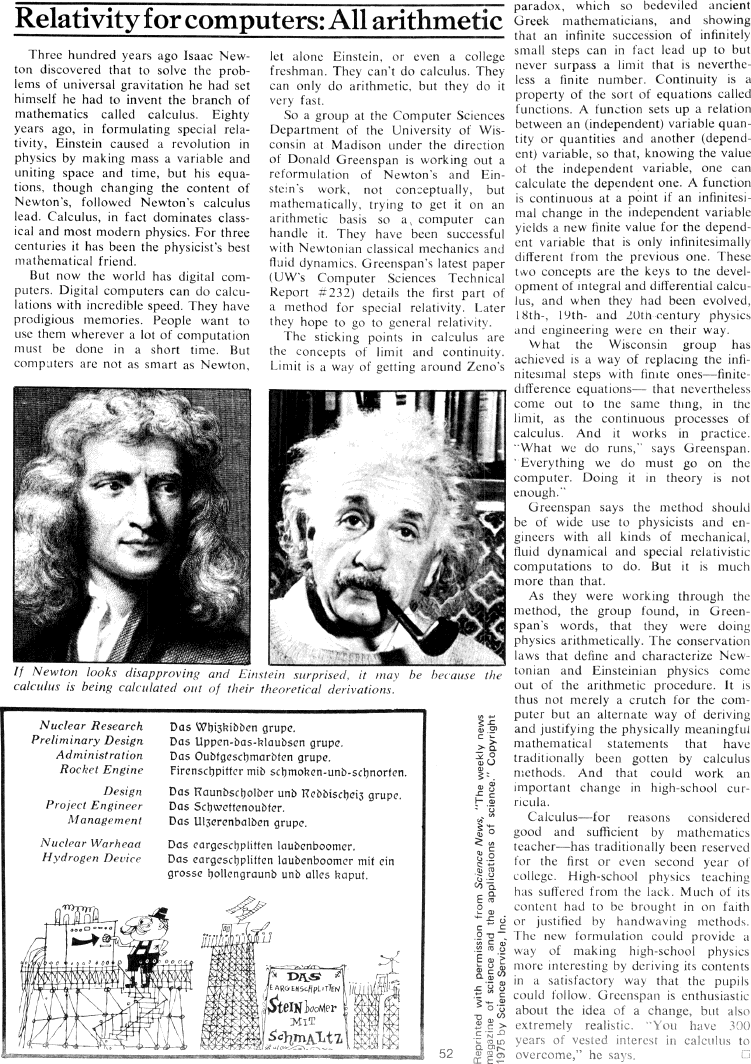The Best of Creative Computing Volume 1 (published 1976)

 `Page 52 << PREVIOUS >> NEXT Jump to page: Go to contents Go to thumbnails`

Relativity for computers: All arithmetic (Mathematic reformulation of Newton's and Einstein's work)```Relativity for computers: All arithmetic
***
Three hundred years ago Isaac Newton discovered that to solve the problems of
himself he had to invent the branch of
mathematics called calculus. Eighty
years ago, in formulating special relativity, Einstein caused a revolution in
physics by making mass a variable and
uniting space and time, but his equations, though changing the content of
Newton's, followed Newton's calculus
lead. Calculus, in fact dominates classical and most modern physics. For three
centuries it has been the physicist's best
mathematical friend.

But now the world has digital computers. Digital computers can do calculations
with incredible speed. They have prodigious memories. People want to
use them wherever a lot of computation
must be done in a short time. But
computers are not as smart as Newton,[image](If Newton looks disapproving and
Einstein surprised, it may be because the calculus is being calculated out of
their theoretical derivations.)
let alone Einstein, or even a college
freshman. They can't do calculus. They
can only do arithmetic, but they do it
very fast.

So a group at the Computer Sciences
Department of the University of Wisconsin at Madison under the direction
of Donald Greenspan is working out a
reformulation of Newton's and Einstein's work, not conceptually, but
mathematically, trying to get it on an
arithmetic basis so a computer can
handle it. They have been successful
with Newtonian classical mechanics and
fluid dynamics. Greenspan's latest paper
(UW's Computer Sciences Technical
Report #232) details the first part of
a method for special relativity. Later
they hope to go to general relativity.

The sticking points in calculus are
the concepts of limit and continuity.

Limit is a way of getting around Zeno's [image]
Greek mathematicians, and showing
that an infinite succession of infinitely
small steps can in fact lead up to but
never surpass a limit that is nevertheless a finite number. Continuity is a
property of the sort of equations called
functions. A function sets up a relation
between an (independent) variable quantity or quantities and another (dependent)
variable, so that, knowing the value of the independent variable, one can
calculate the dependent one. A function
is continuous at a point if an infinitesimal change in the independent variable
yields a new finite value for the dependent variable that is only
infinitesimally
different from the previous one. These
two concepts are the keys to tne development of integral and differential
calculus, and when they had been evolved, l8th-, l9th- and 2Oth century physics
and engineering were on their way.

What the Wisconsin group has
achieved is a way of replacing the infinitesimal steps with finite
ones-finitedifference equations- that nevertheless come out to the same thing,
in the
limit, as the continuous processes of
calculus. And it works in practice.

"What we do runs," says Greenspan.

"Everything we do must go on the
computer. Doing it in theory is not
enough."

Greenspan says the method should
be of wide use to physicists and engineers with all kinds of mechanical,
fluid dynamical and special relativistic
computations to do. But it is much
more than that.

As they were working through the
method, the group found, in Greenspan's words, that they were doing
physics arithmetically. The conservation
laws that define and characterize Newtonian and Einsteinian physics come
out of the arithmetic procedure. It is
thus not merely a crutch for the computer but an alternate way of deriving
and justifying the physically meaningful
mathematical statements that have
methods. And that could work an
important change in high-school curricula.

Calculus-for reasons considered
good and sufficient by mathematics
for the first or even second year of
college. High-school physics teaching
has suffered from the lack. Much of its
content had to be brought in on faith
or justified hy handwaving methods.

The new formulation could provide a
way of making high-school physics
more interesting by deriving its contents
in a satisfactory way that the pupils
could follow. Greenspan is enthusiastic
about the idea of a change, but also
extremely realistic. "You have 300
years of vested interest in calculus to
overcome," he says.

Page 52        << PREVIOUS        >> NEXT        Jump to page:                Go to contents        Go to thumbnails

<!--